In :
import sys as sys
import numpy as np
import pylab as pb
In :
# Loading in data: the 0:1 and 1:2 ensure we get column vectors
# for x and y.
olympics = np.genfromtxt('olympicMarathonTimes.csv', delimiter=',')
x = olympics[:, 0:1]
y = olympics[:, 1:2]
In :
print(x)
print(y)
[[ 1896.]
[ 1900.]
[ 1904.]
[ 1908.]
[ 1912.]
[ 1920.]
[ 1924.]
[ 1928.]
[ 1932.]
[ 1936.]
[ 1948.]
[ 1952.]
[ 1956.]
[ 1960.]
[ 1964.]
[ 1968.]
[ 1972.]
[ 1976.]
[ 1980.]
[ 1984.]
[ 1988.]
[ 1992.]
[ 1996.]
[ 2000.]
[ 2004.]
[ 2008.]
[ 2012.]]
[[ 4.47083333]
[ 4.46472926]
[ 5.22208333]
[ 4.15467867]
[ 3.90331675]
[ 3.56951267]
[ 3.82454477]
[ 3.62483707]
[ 3.59284275]
[ 3.53880792]
[ 3.67010309]
[ 3.39029111]
[ 3.43642612]
[ 3.20583007]
[ 3.13275665]
[ 3.32819844]
[ 3.13583758]
[ 3.0789588 ]
[ 3.10581822]
[ 3.06552909]
[ 3.09357349]
[ 3.16111704]
[ 3.14255244]
[ 3.08527867]
[ 3.10265829]
[ 2.99877553]
[ 3.03392977]]
In :
pb.plot(x, y, 'rx')
Out: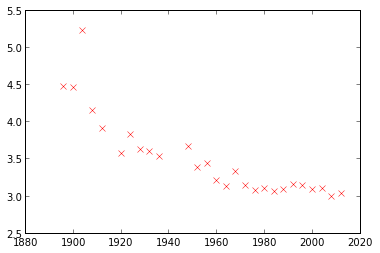In :
m = -0.4
c = 80
In :
# This is an iterative solution for
# finding the gradient and bias.
# Notice how slow it is!
xTest = np.linspace(1890, 2020, 130)[:, None]
for i in arange(10000):
m = ((y - c)*x).sum()/(x*x).sum()
c = (y-m*x).sum()/y.shape
pb.plot(xTest, m*xTest + c, 'b-')
pb.plot(x, y, 'rx')
Out:
[<matplotlib.lines.Line2D at 0x9ac5110>]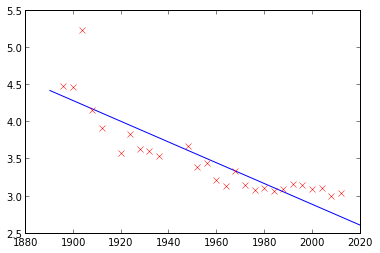In :
print(m)
print(c)
-0.0139467136584
30.7851569022
In :
# Now we will do a quadratic fit.
# First, create a matrix of basis functions in the form of a design matrix
Phi = np.hstack([np.ones(x.shape), x, x**2])
In :
# This is the multivariate linear regression solution.
w = np.linalg.solve(np.dot(Phi.T, Phi), np.dot(Phi.T, y))
print(w)
[[  6.43641952e+02]
[ -6.42502986e-01]
[  1.61109703e-04]]
In :
# For plotting the result create a vector of 'test' inputs and the corresponding basis set
xTest = np.linspace(1890, 2020, 130)[:, None]
PhiTest = np.hstack([np.ones(xTest.shape), xTest, xTest**2])
In :
# Now use the learnt parameters to compute quadratic outputs at test locations
fTest = np.dot(PhiTest, w)
In :
# Plot the data and the model fit.
pb.plot(x, y, 'rx')
pb.plot(xTest, fTest, '-')
Out:
[<matplotlib.lines.Line2D at 0x9ae2d50>]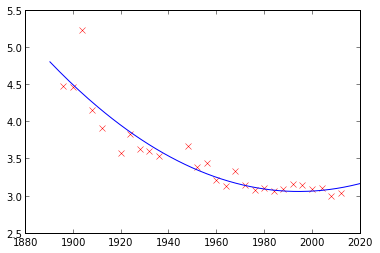In :
# Now we are going to fit a Gaussian process using GPy
import GPy
In :
# First define the covariance function. We'll use the exponentiated quadratic
# First argument is the dimensionality of the input, other arguments are the parameters
kernel = GPy.kern.rbf(1,variance=1.,lengthscale=1.)
In :
# We can get help about GPy kernels with:
kernel?
# We can see what our covariance function is using print
print(kernel)
Name        |  Value   |  Constraints  |  Ties
-------------------------------------------------------
rbf_variance    |  1.0000  |               |
rbf_lengthscale  |  1.0000  |               |
In :
# Now we create a GP regression model using our data and the covariane function
model =GPy.models.GPRegression(x,y,kernel)
In :
# Again we can print the model. We get the log likelihood and parameters.
print(model)
Log-likelihood: -1.188e+02

Name        |  Value   |  Constraints  |  Ties  |  Prior
-----------------------------------------------------------------
rbf_variance    |  1.0000  |     (+ve)     |        |
rbf_lengthscale  |  1.0000  |     (+ve)     |        |
noise_variance   |  1.0000  |     (+ve)     |        |
In :
# The plot command provides a quick way of seeing the model fit.
# In this case the paremters are poor: the length scale is too low (for example)
model.plot()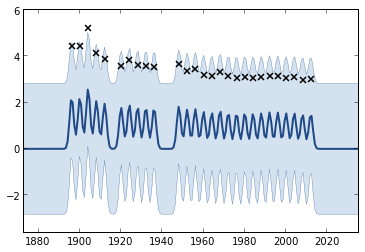In :
# Now we fit the model by maximum likelihood and replot
# The length scale increases considerably
model.optimize()
model.plot()
print(model)
Log-likelihood: -7.790e+00

Name        |   Value   |  Constraints  |  Ties  |  Prior
------------------------------------------------------------------
rbf_variance    |  9.8062   |     (+ve)     |        |
rbf_lengthscale  |  78.8045  |     (+ve)     |        |
noise_variance   |  0.0478   |     (+ve)     |        |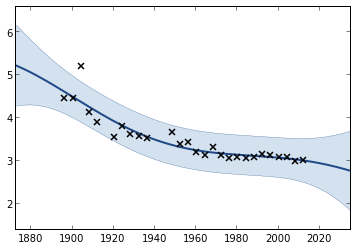In :
# Let's try a different covariance function. This time we try a combination of
# two exponentiated quadratics and a bias (which allows an offset to be inferred).
kernel2 = GPy.kern.rbf(1, lengthscale=4, variance=12)
kernel2 += GPy.kern.rbf(1, lengthscale=20, variance=12)
kernel2 += GPy.kern.bias(1)
In :
# Build the model using this covariance function
model2 = GPy.models.GPRegression(x,y,kernel2)
model2.plot()
print(model2)
Log-likelihood: -5.816e+01

Name         |   Value   |  Constraints  |  Ties  |  Prior
--------------------------------------------------------------------
rbf_2_variance    |  12.0000  |     (+ve)     |        |
rbf_2_lengthscale  |  4.0000   |     (+ve)     |        |
rbf_1_variance    |  12.0000  |     (+ve)     |        |
rbf_1_lengthscale  |  20.0000  |     (+ve)     |        |
bias_variance    |  1.0000   |     (+ve)     |        |
noise_variance    |  1.0000   |     (+ve)     |        |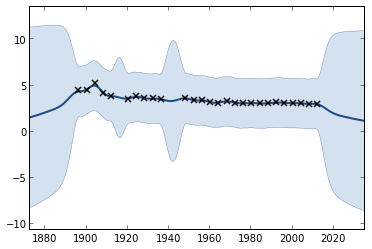In :
# Now optimize the new model
model2.optimize()
model2.plot()
print(model2)
Log-likelihood: -6.160e+00

Name         |   Value   |  Constraints  |  Ties  |  Prior
--------------------------------------------------------------------
rbf_2_variance    |  0.0217   |     (+ve)     |        |
rbf_2_lengthscale  |  6.7619   |     (+ve)     |        |
rbf_1_variance    |  1.0682   |     (+ve)     |        |
rbf_1_lengthscale  |  70.1276  |     (+ve)     |        |
bias_variance    |  18.7005  |     (+ve)     |        |
noise_variance    |  0.0381   |     (+ve)     |        |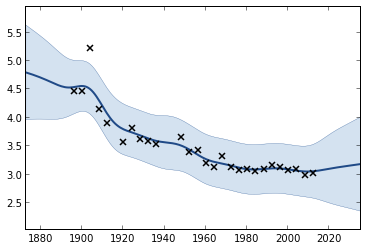In :
# GPy allows us to set constraints on parameters.
# These commands use regular expressions to constrain
# particular parameter values.
model.unconstrain('rbf.*variance')
model.constrain_bounded('.*variance',.0001,5.)
model.constrain_bounded('.*len',.002,10.)
model.constrain_fixed('bias.*var',40.)
model.optimize()
print(model)
model.plot()
Warning: re-constraining these parameters
noise_variance
Warning: changing parameters to satisfy constraints
Warning: re-constraining these parameters
rbf_lengthscale
Warning: changing parameters to satisfy constraints

Log-likelihood: -2.665e+01

Name        |  Value   |  Constraints   |  Ties  |  Prior
------------------------------------------------------------------
rbf_variance    |  4.9367  |  (0.0001,5.0)  |        |
rbf_lengthscale  |  9.9990  |  (0.002,10.0)  |        |
noise_variance   |  0.0346  |  (0.0001,5.0)  |        |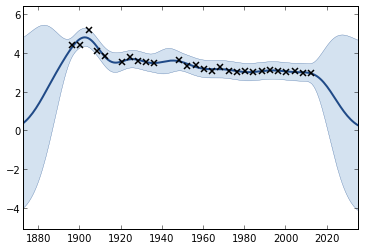In :
# We can make predictions from the Gaussian process using model.predict.
# Here, we first place those predictions input locations in an array.
xfuture = np.array([2015,2030,2045,2060])[:,None]
mean,var,q1,future = np.array([2015,2030,2045,2060])[:,None]
mean,var,q1,q3 = model2.predict(xfuture)
print(mean)
[[ 3.07209821]
[ 3.15954177]
[ 3.2398172 ]
[ 3.32644406]]
In :
# We can also plot the form of covariance functions we use
k = GPy.kern.rbf(1,variance=1.,lengthscale=0.2)
print(k)
k.plot()
Name        |  Value   |  Constraints  |  Ties
-------------------------------------------------------
rbf_variance    |  1.0000  |               |
rbf_lengthscale  |  0.2000  |               |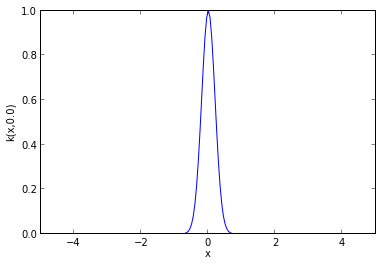In :
print(model.kern)
model.kern.plot()
Name        |  Value   |  Constraints  |  Ties
-------------------------------------------------------
rbf_variance    |  4.9367  |               |
rbf_lengthscale  |  9.9990  |               |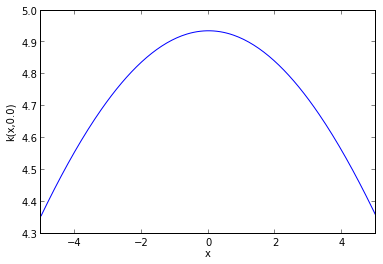In :
#Here we show the affect of changing the variance on the
#covariance function.
variances = [.5,1.,2.,4.]
for v in variances:
k = GPy.kern.rbf(1,variance=v,lengthscale = .2)
k.plot()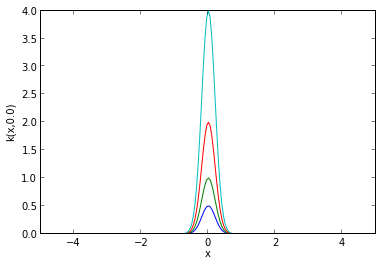In :